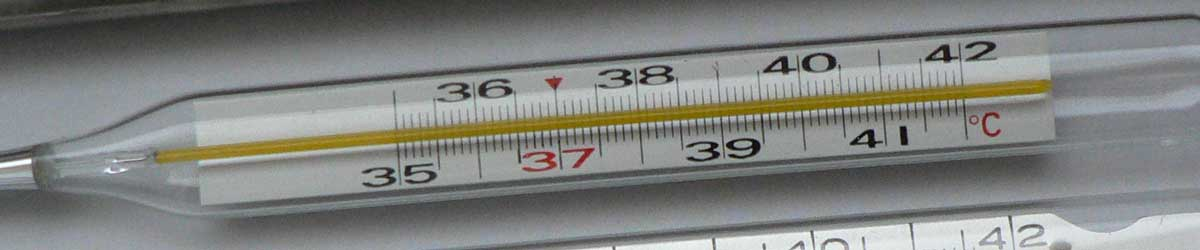# Delisle to Newton Conversion

Enter the temperature in Delisle below to get the value converted to Newton.

Results in Degrees Newton:1 °De = 32.78 °N
Do you want to convert Newton to Delisle?

## How to Convert Delisle to NewtonConvert degrees Delisle to degrees Newton with this simple formula:

degrees Newton = 33 - [°De] × 11 / 50

Insert the [°De] temperature measurement in the formula and then solve to find the result.

For example, let's convert 50 °De to degrees Newton:

50 °De = ( 33 - 50 × 11 / 50 ) = 22 °N

## Delisle

The Delisle scale is a temperature scale that sets a fixed zero point equal to the temperature of boiling water. The Delisle scale is uses incrementing degrees as the temperature decreases. It runs from 0 degrees at the boiling point of water to 150 degrees at the the freezing point.

Delisle can be abbreviated as De; for example, 1 degree Delisle can be written as 1 °De.

### Important Temperatures In Degrees Delisle

TemperatureDegrees Delisle
Absolute Zero559.725 °De
Freezing Point of Water150 °De
Boiling Point of Water0 °De

## Newton

The Newton scale is a temperature scale that sets a fixed zero point equal to the freezing point water. The scale uses both arithmetic progression and geometric progression. The scale defines 18 reference points and the corresponding "degrees of heat" and geometric progressions.

Newton can be abbreviated as N; for example, 1 degree Newton can be written as 1 °N.

## Delisle to Newton Conversion Table

Delisle measurements converted to Newton
Delisle Newton
-50 °De 44 °N
-40 °De 41.8 °N
-30 °De 39.6 °N
-20 °De 37.4 °N
-10 °De 35.2 °N
0 °De 33 °N
10 °De 30.8 °N
20 °De 28.6 °N
30 °De 26.4 °N
40 °De 24.2 °N
50 °De 22 °N
60 °De 19.8 °N
70 °De 17.6 °N
80 °De 15.4 °N
90 °De 13.2 °N
100 °De 11 °N
110 °De 8.8 °N
120 °De 6.6 °N
130 °De 4.4 °N
140 °De 2.2 °N
150 °De 0 °N
160 °De -2.2 °N
170 °De -4.4 °N
180 °De -6.6 °N
190 °De -8.8 °N
200 °De -11 °N
300 °De -33 °N
400 °De -55 °N
500 °De -77 °N
600 °De -99 °N
700 °De -121 °N
800 °De -143 °N
900 °De -165 °N
1,000 °De -187 °N